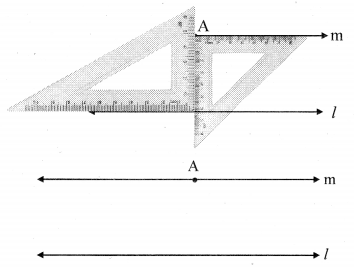# Maharashtra Board Class 8 Maths Solutions Chapter 2 Parallel Lines and Transversals Practice Set 2.3

## Maharashtra State Board Class 8 Maths Solutions Chapter 2 Parallel Lines and Transversals Practice Set 2.3

Question 1.
Draw a line l. Take a point A outside the line. Through point A draw a line parallel to line l.
Solution:
Steps of construction:

1. Draw a line l and take any point A outside the line.
2. Place a set-square, such that one arm of the right angle passes through A and the other arm is on line l.
3. Place the second set-square as shown in the figure such that the vertex of the right angle is at point A.
4. Hold the two set-squares in place and draw a line parallel to line l through the edge of the second set-square. Name the line as m.Line m is the required line parallel to line l and passing through point A.

Question 2.
Draw a line l. Take a point T outside the line. Through point T draw a line parallel to line l.
Solution:
Steps of construction:

1. Draw a line l and take any point T outside the line.
2. Place a set-square, such that one arm of the right angle passes through T and the other arm is on line l.
3. Place the second set-square as shown in the figure such that the vertex of the right angle is at point T.
4. Hold the two set-squares in place and draw a line parallel to line l through the edge of the second set-square. Name the line as m.Line m is the required line parallel to line l and passing through point T.

Question 3.
Draw a line m. Draw a line n which is parallel to line m at a distance of 4 cm from it.
Solution:
Steps of construction:

1. Draw a line m and take any two points M and N on the line.
2. Draw perpendiculars to line m at points M and N.
3. On the perpendicular lines take points S and T at a distance 4 cm from points M and N respectively.
4. Draw a line through points S and T. Name the line as n.Line n is parallel to line m at a distance of 4 cm from it.